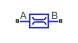# Laminar Leakage (IL)

Isothermal liquid element that models laminar leakage flow for various geometries

Since R2020a

•Libraries:
Simscape / Foundation Library / Isothermal Liquid / Elements

## Description

The Laminar Leakage (IL) block models laminar flow in isothermal liquid networks. The block includes several common flow passage geometries and a custom geometry. Select a Cross-sectional geometry parameter value and then specify other parameter values based on the selected option:

• `Circular` — Provide the diameter and longitudinal length.

• `Annular` — Provide the inner diameter, outer diameter, and longitudinal length.

• `Rectangular` — Provide the width, height, and longitudinal length.

• `Elliptical` — Provide the major axis, minor axis, and longitudinal length.

• `Equilateral triangular` — Provide the length of the triangle side and longitudinal length.

• `Custom` — Provide the flow resistance value, that is, the ratio of pressure loss to mass flow rate. In this configuration, the block acts as a linear hydraulic resistance.

The flow rate calculations are based on the Hagen-Poiseuille equation, which is valid for fully developed laminar flow:

`${K}_{\text{geom}}=\left\{\begin{array}{ll}\frac{\pi {d}^{4}}{128},\hfill & \text{circular}\hfill \\ \frac{\pi }{128}\left({d}_{o}^{4}-{d}_{i}^{4}-\frac{{\left({d}_{o}^{2}-{d}_{i}^{2}\right)}^{2}}{\mathrm{log}\left({d}_{o}/{d}_{i}\right)}\right),\hfill & \text{annular}\hfill \\ \frac{w{h}^{3}}{12}\left(1-\frac{192h}{w{\pi }^{5}}\mathrm{tanh}\left(\frac{\pi w}{2h}\right)\right),\hfill & \text{rectangular}\hfill \\ \frac{\pi {\left(ab\right)}^{3}}{64\left({a}^{2}+{b}^{2}\right)},\hfill & \text{elliptical}\hfill \\ {l}_{side}^{4}\frac{\sqrt{3}}{320},\hfill & \text{triangular}\hfill \end{array}$`
`$\overline{\rho }=\frac{{\rho }_{A}+{\rho }_{B}}{2}$`

where:

• $\stackrel{˙}{m}$A is the mass flow rate through port A.

• pA and pB are pressures at ports A and B, respectively.

• Kgeom is the coefficient calculated based on the cross-sectional geometry.

• d is the diameter of the circular cross section.

• do is the outer diameter of the annular cross section.

• di is the inner diameter of the annular cross section.

• w is the width of the rectangular cross section.

• h is the height of the rectangular cross section.

• a is the major axis of the elliptical cross section.

• b is the minor axis of the elliptical cross section.

• lside is the length of the side of the triangular cross section.

• L is the longitudinal length.

• R is the linear hydraulic resistance for custom geometry.

• ν is the liquid kinematic viscosity at atmospheric pressure, which is a global parameter defined by the Isothermal Liquid Properties (IL) block connected to the circuit.

• $\overline{\rho }$ is the average fluid mixture density.

• ρA and ρB are fluid mixture density values at ports A and B, respectively. Equations used to compute the fluid mixture density depend on the selected isothermal liquid model. For detailed information, see Isothermal Liquid Modeling Options.

## Ports

### Conserving

expand all

Isothermal liquid conserving port associated with the inlet or outlet of the laminar leakage. This block has no intrinsic directionality.

Isothermal liquid conserving port associated with the inlet or outlet of the laminar leakage. This block has no intrinsic directionality.

## Parameters

expand all

Select a cross-sectional shape and then specify other parameter values based on the selected option.

Diameter of the circular cross section.

#### Dependencies

Enabled when the Cross-sectional geometry parameter is set to `Circular`.

Inner diameter of the annular cross section.

#### Dependencies

Enabled when the Cross-sectional geometry parameter is set to `Annular`.

Outer diameter of the annular cross section.

#### Dependencies

Enabled when the Cross-sectional geometry parameter is set to `Annular`.

Width of the rectangular cross section.

#### Dependencies

Enabled when the Cross-sectional geometry parameter is set to `Rectangular`.

Height of the rectangular cross section.

#### Dependencies

Enabled when the Cross-sectional geometry parameter is set to `Rectangular`.

Major axis of the elliptical cross section.

#### Dependencies

Enabled when the Cross-sectional geometry parameter is set to `Elliptical`.

Minor axis of the elliptical cross section.

#### Dependencies

Enabled when the Cross-sectional geometry parameter is set to `Elliptical`.

Length of the triangle side for the equilateral triangular cross section.

#### Dependencies

Enabled when the Cross-sectional geometry parameter is set to `Equilateral triangular`.

Length of the flow passage.

Ratio of pressure loss to the mass flow rate. If cross-sectional geometry is not known, the block acts as a linear hydraulic resistance.

#### Dependencies

Enabled when the Cross-sectional geometry parameter is set to `Custom`.

 Manring, N. D., Hydraulic Control Systems. p. 71. Hoboken, New Jersey: John Wiley & Sons, 2005.